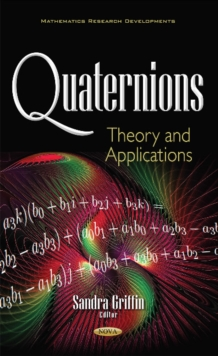Supporting your high street Find out how »
• My AccountQuaternions : Theory & Applications Hardback

Edited by Sandra Griffin

Description

This book focuses on the theory and applications of quaternions.

Chapter One collects some old problems on lattice orders and directed partial orders on complex numbers and quaternions, and summarises recent development in answering those questions.

Chapter Two discusses spin 1 particles with anomalous magnetic moments in the external uniform electric field.

Chapter Three examines techniques of projective operators used to construct solutions for a spin 1 particle with anomalous magnetic moment in the external uniform magnetic field.

Chapter Four analyses the implementation of a cheap Micro AHRS (Attitude and Heading Reference System) using low-cost inertial sensors.

Chapter Five reviews the basic concepts of quaternion and reduced biquaternions algebra.

It introduces the 2D Hermite-Gaussian functions (2D-HGF) as the eigenfunction of discrete quaternion Fourier transform (DQFT) and discrete reduced biquaternion Fourier transform (DRBQFT), and the eigenvalues of two dimensional Hermite-Gaussian functions for three types of DQFT and two types of DRBQFT.

Chapter Six investigates a leader-follower formation control problem of quadrotors.

Chapter Seven considers determinantal representations the Drazin and weighted Drazin inverses over the quaternion skew field.

Information

• Format: Hardback
• Pages: 190 pages
• Publisher: Nova Science Publishers Inc
• Publication Date:
• Category: Algebra
• ISBN: 9781536107685

£90.99

£69.15

on all orders

Pick up orders

from local bookshops